NEET  >  Continuous Charge Distribution

# Continuous Charge Distribution Notes | Study Physics Class 12 - NEET

## Document Description: Continuous Charge Distribution for NEET 2022 is part of Physics Class 12 preparation. The notes and questions for Continuous Charge Distribution have been prepared according to the NEET exam syllabus. Information about Continuous Charge Distribution covers topics like and Continuous Charge Distribution Example, for NEET 2022 Exam. Find important definitions, questions, notes, meanings, examples, exercises and tests below for Continuous Charge Distribution.

Introduction of Continuous Charge Distribution in English is available as part of our Physics Class 12 for NEET & Continuous Charge Distribution in Hindi for Physics Class 12 course. Download more important topics related with notes, lectures and mock test series for NEET Exam by signing up for free. NEET: Continuous Charge Distribution Notes | Study Physics Class 12 - NEET
 1 Crore+ students have signed up on EduRev. Have you?

INTRODUCTION TO CONTINUOUS CHARGE DISTRIBUTION
With the help of Coulomb’s Law and Superposition Principle, we can easily find out the electric field due to the system of charges or discrete system of charges. The word discrete means every charge is different and has the existence of its own. Suppose, a system of charges having charges as q1, q2, q3……. up to qn. We can easily find out the net charge by adding charges algebraically and net electric field by using the principle of superposition.
This is because:

• Discrete system of charges is easier to solve
• Discrete system of charges do not involve calculus in calculations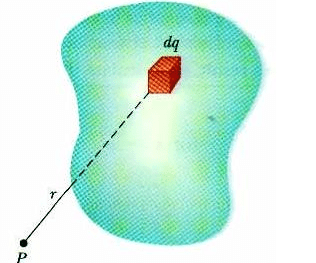Fig: A system in which charge is distributed over a conductor, is called continuous charge distribution system

But how to calculate electrostatics terms in continuous charge system? For an Example if there is a rod with charge q, uniformly distributed over it and we wish to find the electric field at some distance ‘r’ due it. It would be illogical and irrelevant to simply add electric field using principle of superposition as the charge is uniformly distributed over the rod. So we take a small element of the rod and integrate it with proper limits.

We consider element, based on how density of charge is centered on the material or object. If the charge is uniformly distributed over the surface of the conductor, then it is called Surface Density. If the charge varies linearly along the length of the conductor, then it is called Linear Charge Density. And if the charge changes with volume of the conductor, then it is called Volume Charge Density.

WHAT IS CONTINUOUS CHARGE DISTRIBUTION?

##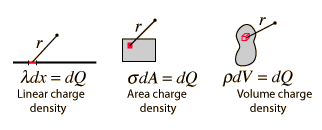Fig: Types of Charge Distribution

The continuous charge distribution system is a system in which the charge is uniformly distributed over the conductor. In continuous charge system, infinite numbers of charges are closely packed and have minor space between them. Unlikely from the discrete charge system, the continuous charge distribution is uninterrupted and continuous in the conductor. There are three types of the continuous charge distribution system.

• Linear Charge Distribution
• Surface Charge Distribution
• Volume Charge Distribution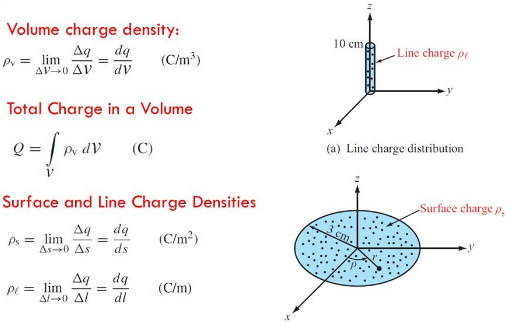Fig: Types of charge distribution system

LINEAR CHARGE DENSITY
When the charge is non-uniformly distributed over the length of a conductor, it is called linear charge distribution. It is also called linear charge density and is denoted by the symbol λ (Lambda).
Mathematically linear charge density is λ = dq/dl
The unit of linear charge density is C/m. If we consider a conductor of length ‘L’ with surface charge density λ and take an element dl on it, then small charge on it will be
dq = λl
So, the electric field on small charge element dq will be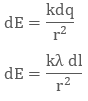To calculate the net electric field we will integrate both sides with proper limit, that is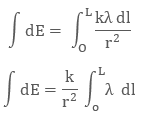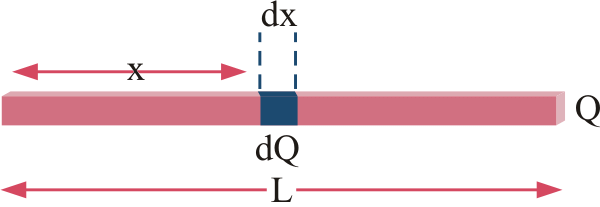Fig: We take small element x and integrate it in case of linear charge density

SURFACE CHARGE DENSITY
When the charge is uniformly distributed over the surface of the conductor, it is called Surface Charge Density or Surface Charge Distribution. It is denoted by the symbol σ (sigma) symbol and is the unit is C/m2.
It is also defined as charge/ per unit area. Mathematically surface charge density is σ = dq/ds
where dq is the small charge element over the small surface ds. So, the small charge on the conductor will be dq = σds
The electric field due to small charge at some distance ‘r’ can be evaluated as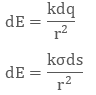Integrating both sides with proper limits we get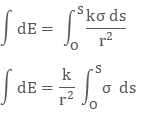VOLUME CHARGE DENSITY
When the charge is distributed over a volume of the conductor, it is called Volume Charge Distribution. It is denoted by symbol ρ (rho). In other words charge per unit volume is called Volume Charge Density and its unit is C/m3. Mathematically, volume charge density is ρ = dq/dv
where dq is small charge element located in small volume dv. To find total charge we will integrate dq with proper limits. The electric field due to dq will be
dq = ρ dv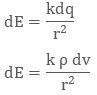Integrating both sides with proper limits we get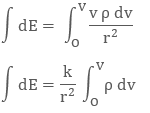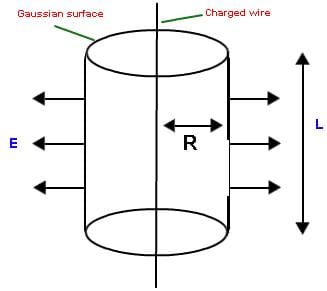`Fig: We can easily find electric field in different geometries using charge distribution system`
The document Continuous Charge Distribution Notes | Study Physics Class 12 - NEET is a part of the NEET Course Physics Class 12.
All you need of NEET at this link: NEET

## Physics Class 12

157 videos|425 docs|213 tests
 Use Code STAYHOME200 and get INR 200 additional OFF

## Physics Class 12

157 videos|425 docs|213 tests

### How to Prepare for NEET

Read our guide to prepare for NEET which is created by Toppers & the best Teachers

Track your progress, build streaks, highlight & save important lessons and more!

,

,

,

,

,

,

,

,

,

,

,

,

,

,

,

,

,

,

,

,

,

;